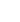15min:
COMPUTATIONAL MOLECULAR SPECTROSCOPY OF FeCN IN THE 6ELECTRONIC GROUND STATE.

TSUNEO HIRANO, REI OKUDA AND UMPEI NAGASHIMA, Research Institute for Computational Sciences, National Institute of Advanced Industrial Science and Technology, 1-1-1 Umezono, Tsukuba, Ibaraki 305-8568, Japan; MICHIKO AMANO, YUKARI MITSUI, SACHIKO S. ITONO, Department of Chemistry, Faculty of Science, Ochanomizu University, 2-1-1 Otsuka, Bunkyo-ku, Tokyo 112-8610, Japan; PER JENSEN, Theoretische Chemie, Bergische Universität, D-42097 Wuppertal, Germany.

We have previously reported a computational molecular spectroscopic study of 6i FeNC,\footnoteT. Hirano, R. Okuda, U. Nagashima, V.~Spirko, and P. Jensen, J. Mol. Spectrosc. , \textbf236, 234-247 (2006). where we showed that the experimentally derived, too-short C-N bond length (r e(C-N) = 1.03(8) Å) can be ascribed to an inadequate treatment of the large amplitude bending motion in the experimental determination of r0. Here, we report analogous calculations for 6i FeCN.

Based on the three-dimensional potential energy surface calculated at the MR-SDCI+Q+E rel/[Roos~ANO (Fe), aug-cc-pVQZ (C, N)] level of theory, the standard spectroscopic parameters of Fe12CN and Fe13CN are derived by perturbation methods, and ro-vibrationally averaged bond lengths \langle r \rangle have been predicted as expectation values obtained with ro-vibrational wavefunctions from the MORBID program. Some of the spectroscopic constants thus determined are: r e(Fe-C) = 2.048 Å and r e(C-N) = 1.168 Å,1=2179 cm-1,2=173 cm-1,3=420 cm-1, dipole moment = 4.59 D, spin-orbit coupling constant A SO=-83 cm-1, \langle r(Fe-C)\rangle0 = 2.082 Å, and \langle r(C-N)\rangle0 = 1.172 Å. In variational MORBID calculations, rovibronic energy levels are determined, and some vibrational bands are simulated. The bending potential is shallow, and the MORBID calculations show that the zero-point averaged structure is bent with the expectation value \langle \angle(Fe-C-N) \rangle0 = 170(5)o (where the number in parentheses is the quantum-mechanical uncertainty). We compare the 6i FeCN results with those obtained for 6i FeNC. Since there are no experimental spectroscopic data available for FeCN, we hope that the predictions made here may be useful in the experimental investigation of this molecule.